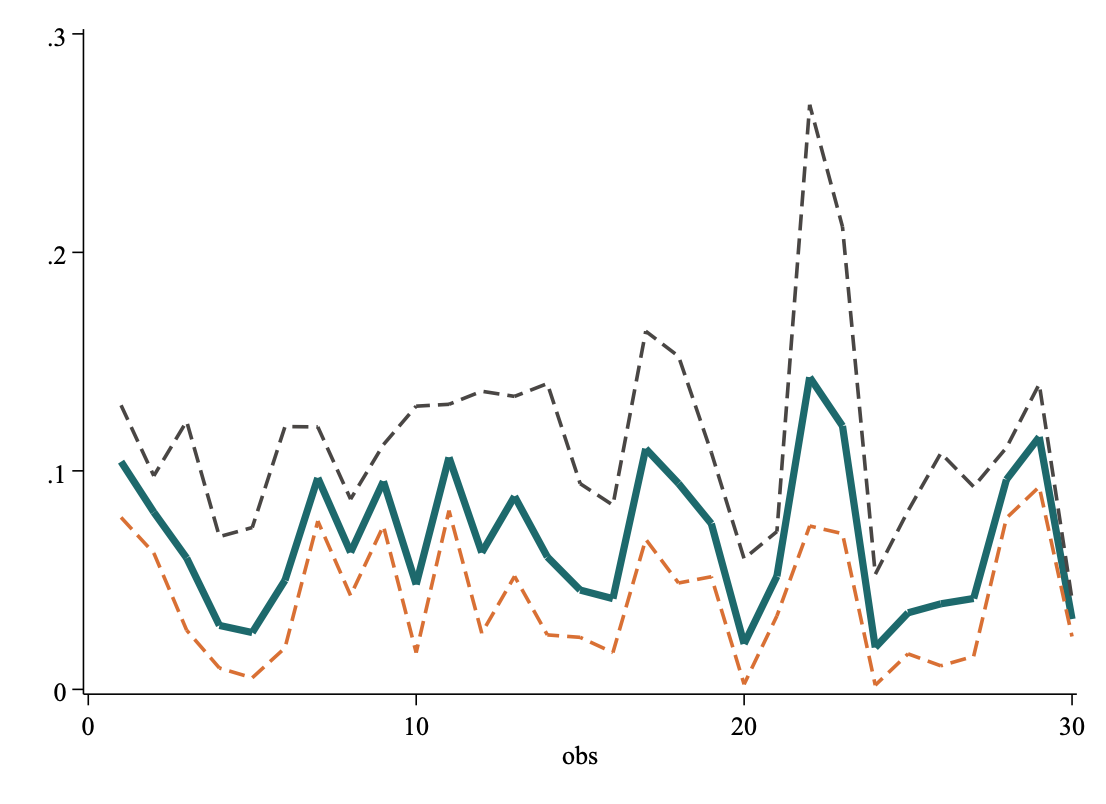# Find Semi-max Density Values in Normal Distribution

Today afternoon, a friend asked me a question, I can’t describe this question in English accurately, but I will try my best. Now, she has 30 sequences, each has 10 thousand numeric elements, they seemingly follow a normal distribution. For a normal distribution, the max density value correspond to mean. she want to know at which value, the density value equals half of the max density value.

The original data is: A.csv，each line is a sequence. First we need to make a tranposition:

She gives me 30 sequences, each have 10,000 observations. For example, for the fist sequence, the kenel density distribution is:From above diagrams, we can see at about 0.1, the density get its biggest value, about 17. What we want to know is at which value, the density value is about 17/2 = 8.5. Obviously, there are two values meet this requirement.

My idea is to first get 10000 lattice points of the distribution by kernel density estimation, then sort the density value of each lattice point, find the largest divided by 2, and then find the two lattice points closest to the semi-max value. The code is:These two points are exactly what we needed!

Next, program a loop to get all 30 sequences’ semi-max values:

A2.csv is like this:

semimaxv1 semimaxv2 semimaxd1 semimaxd2 max maxd
.078701444 .1300306 9.1357012 9.1339693 .10422645 18.27581
.06238056 .097713493 13.26233 13.267978 .081068322 26.527439
.027084824 .12270609 4.3110609 4.3171973 .060132872 8.6377687
.0097687775 .069909528 6.2074771 6.1685586 .02927785 12.341722
.0052797976 .074061677 5.0783057 5.0829434 .026011925 10.177743
.018993862 .12027971 4.0163946 4.0077281 .049942315 8.011528
.077044569 .1201292 10.900649 10.90671 .09677574 21.811472
.042942528 .087064542 10.498396 10.50073 .062700093 21.005436
.074877165 .11197978 12.780385 12.78516 .095224872 25.565735
.016825253 .12961259 3.506664 3.5183191 .048007637 7.0338864

To make sure the answers are correct, we can plot two comparasion charts:Now, I’m sure these answers are correct!